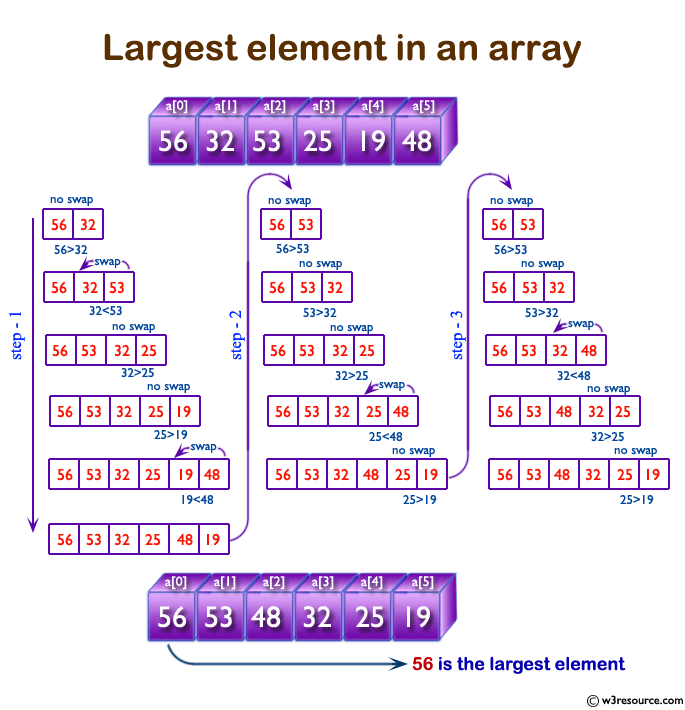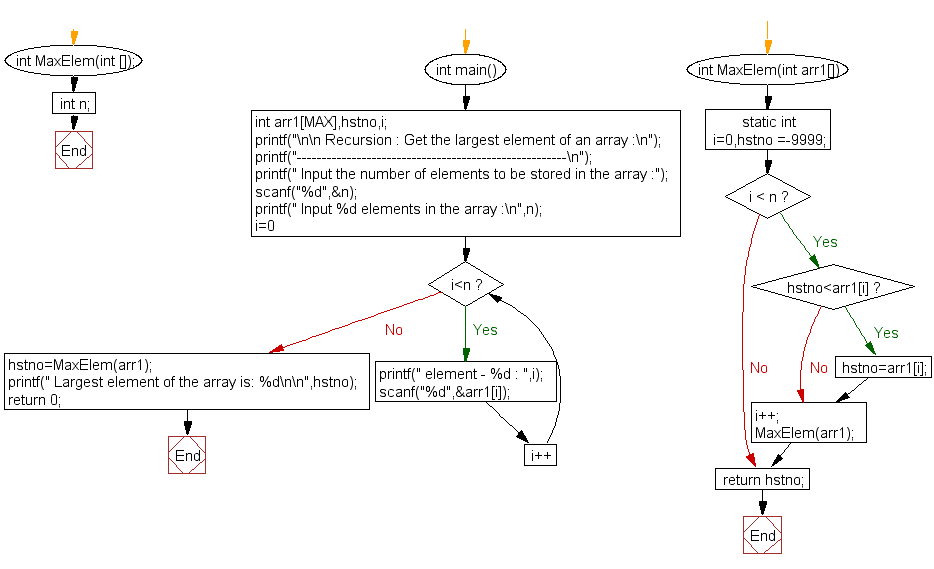﻿ C Program: Get the largest element of an array - w3resource

# C Exercises: Get the largest element of an array

## C Recursion: Exercise-8 with Solution

Write a program in C to get the largest element of an array using recursion.

Pictorial Presentation:Sample Solution:

C Code:

``````#include<stdio.h>
#define MAX 100

int MaxElem(int []);
int n;

int main()
{
int arr1[MAX],hstno,i;
printf("\n\n Recursion : Get the largest element of an array :\n");
printf("------------------------------------------------------\n");

printf(" Input the number of elements to be stored in the array :");
scanf("%d",&n);

printf(" Input %d elements in the array :\n",n);
for(i=0;i<n;i++)
{
printf(" element - %d : ",i);
scanf("%d",&arr1[i]);
}
hstno=MaxElem(arr1);//call the function MaxElem to return the largest element
printf(" Largest element of the array is: %d\n\n",hstno);
return 0;
}
int MaxElem(int arr1[])
{
static int i=0,hstno =-9999;
if(i < n)
{
if(hstno<arr1[i])
hstno=arr1[i];
i++;
MaxElem(arr1);//calling the function MaxElem itself to compare with further element
}
return hstno;
}
```
```

Sample Output:

``` Recursion : Get the largest element of an array :
------------------------------------------------------
Input the number of elements to be stored in the array :5
Input 5 elements in the array :
element - 0 : 5
element - 1 : 10
element - 2 : 15
element - 3 : 20
element - 4 : 25
Largest element of the array is: 25
```

Explanation:

```int MaxElem(int arr1[])
{
static int i=0,hstno =-9999;
if(i < n)
{
if(hstno<arr1[i])
hstno=arr1[i];
i++;
MaxElem(arr1);//calling the function MaxElem itself to compare with further element
}
return hstno;
}
```

The function MaxElem() takes an integer array arr1 as input and finds the maximum element of the array using recursion.

The function initializes a static variable i to 0 and hstno to a very small negative number (-9999) to handle cases where all elements in the array are negative. It then checks if the index i is less than the length of the array n. If it is, the function checks if the current element arr1[i] is greater than the current maximum 'hstno'. If it is, the maximum is updated to the current element. The index i is then incremented and the function is called recursively with the same array to compare with the next element.

Once the index i becomes equal to the length of the array, the function returns the maximum element 'hstno'.

Time complexity and space complexity:

The time complexity of this function is O(n), where n is the number of elements in the array. This is because the function needs to traverse the entire array once to find the maximum element.

The space complexity of this function is O(1), because it uses a constant amount of memory for the integer variables ‘i’ and ‘hstno’. The function does not use any extra memory proportional to the size of the input array.

Flowchart:C Programming Code Editor:

Have another way to solve this solution? Contribute your code (and comments) through Disqus.

What is the difficulty level of this exercise?

Test your Programming skills with w3resource's quiz.

﻿

## C Programming: Tips of the Day

__FILE__ macro shows full path

```#include <string.h>

#define __FILENAME__ (strrchr(__FILE__, '/') ? strrchr(__FILE__, '/') + 1 : __FILE__)
For Windows use '\\' instead of '/'.
```

Ref : https://bit.ly/3iEWRoT

We are closing our Disqus commenting system for some maintenanace issues. You may write to us at reach[at]yahoo[dot]com or visit us at Facebook